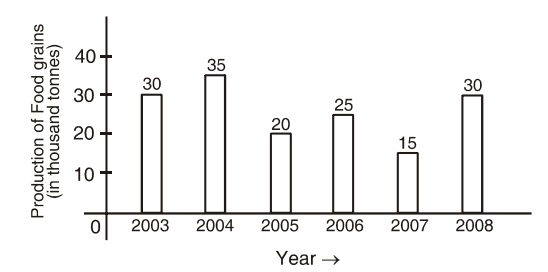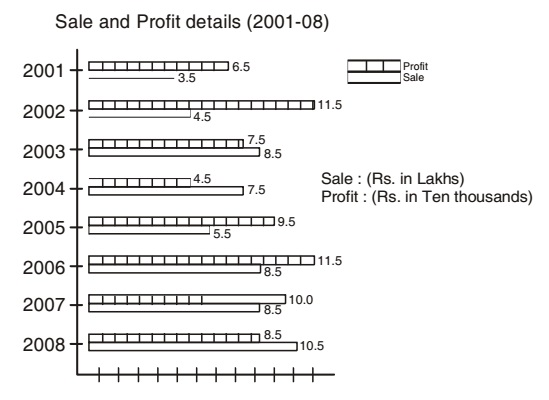## Bar graph

#### Data Interpretation

Direction: The following bar graph shows the producation of food grains in India during certain year. Study the graph carefully and answer the questions given below:1. The difference between the foodgrain productions of 2004 and 2008 is:

1. Differnce between the production of foodgrains during 2004 and 2008 = 35 - 30 =5 thousand tonnes.

##### Correct Option: C

Differnce between the production of foodgrains during 2004 and 2008 = 35 - 30 =5 thousand tonnes.

1. Percentage increase in the production of foodgrains in the year 2008 over the year 2007 is:

1. Percentage increase in food grain production = (30 - 15 )/15 x 100

##### Correct Option: D

Percentage increase in food grain production = (30 - 15 )/15 x 100 = 100%

1. Total food grain production in 2005 and 2007 is equal to the production during which of the following years?

1. Total production in 2005 and 2007 = 20 + 15 = 35 thousand tonnes.

##### Correct Option: B

Total production in 2005 and 2007 = 20 + 15 = 35 thousand tonnes. This total production is equal to the production in the year 2004.

1. Rate of change in quantity of production in which of the following two consecutive years is at the minimum level?

1. Rate of change in the amount of production in the year 2004 over the year 2003 = (Production in 2004 - Production in 2003 ) x 100/ Production in 2003
Similarly
Rate of change in the amount of production in the year 2006 over the year 2005 = (Production in 2006 - Production in 2005 ) x 100/ Production in 2005

##### Correct Option: A

Rate of change in the amount of production in the year 2004 over the year 2003 = (Production in 2004 - Production in 2003 ) x 100/ Production in 2003
Rate of change in the amount of production in the year 2004 over the year 2003 = (35 - 30) x 100 / 30 = 5 x 100 / 30 = 16.67%
Similarly
Rate of change in the amount of production in the year 2006 over the year 2005 = (25 - 20) x 100 / 20 = 5 x 100/20 = 25%
Rate of change in the amount of production in the year 2007 over the year 2008 = (30 - 15) x 100 / 15 = 15 x 100/15= 100%
Rate of change in the amount of production in the year 2006 over the year 2007 = (25 - 15) x 100 / 15 = 10 x 100/15= 66.67%
Hence, it is clear that the rate of change in the amount of production during the consecutive years 2007 and 2008 is at the highest level.

Direction: The bar graph as shows below gives information about the sale and profit details of a departmental store during the years from 2001 - 2008. Study the graph carefully and answer the questions asked here under.1. Mean of annual increase in sale from 2003 to 2008 (rupees in lakhs) is:

1. Mean of annual increase in sale from 2003 to 2008 = (Sale in 2008 - sale in 2003 )/ (Difference in years)

##### Correct Option: D

Mean of annual increase in sale from 2003 to 2008 = (Sale in 2008 - sale in 2003 )/ (Difference in years)
Mean of annual increase in sale from 2003 to 2008 = 10.5 - 8.5/ 5 = 0.4